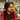# BST Construction

## Properties of BST:

• Binary tree
• Value of root node is greater than its left child and less than its right child

## A tree Node:

``````var TreeNode = function (val) {
this.val = val
this.left = null
this.right = null
}``````

## Naive approach to construct a BST:

``````var root = new TreeNode(1)
root.left = new TreeNode(0)
root.right = new TreeNode(2)
root.left.left = new TreeNode(3)
root.right.right = new TreeNode(4)``````

## Constructing BST from an array representing a tree:

Consider a tree:

``````           5
/     \
2       9
/     \      \
1     3       10``````

The above tree can be represented in an array like:

``[5, 2, 9, 1, 3, null, 10]``

### Explanation:

• The array starts with the root element 5, position i=0.
• Any node’s left element = 2*i + 1
• Any node’s right element = 2*i + 2
• null value represents that the left child of 9 is empty.

### Constructing a tree from this array:

``````function constructTree(arr, i) {
if (i >= arr.length) return null

var node = new TreeNode(arr[i])
node.left = constructTree(arr, 2 * i + 1)
node.right = constructTree(arr, 2 * i + 2)

return node
}

var root = constructTree([5, 2, 9, 1, 3, null, 10], 0)``````Written by Gagandeep Rangi who likes to talk about himself in third person. Twitter Instagram